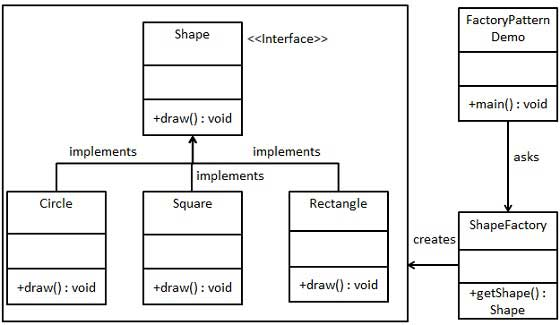# 工厂模式

2021-11-26 09:40 更新

## 实现

FactoryPatternDemo，我们的演示类使用 ShapeFactory 来获取 Shape 对象。它将向 ShapeFactory 传递信息（CIRCLE / RECTANGLE / SQUARE），以便获取它所需对象的类型。### 步骤 1

Shape.java

``````public interface Shape {
void draw();
}``````

### 步骤 2

Rectangle.java

``````public class Rectangle implements Shape {

@Override
public void draw() {
System.out.println("Inside Rectangle::draw() method.");
}
}``````

Square.java

``````public class Square implements Shape {

@Override
public void draw() {
System.out.println("Inside Square::draw() method.");
}
}``````

Circle.java

``````public class Circle implements Shape {

@Override
public void draw() {
System.out.println("Inside Circle::draw() method.");
}
}``````

### 步骤 3

ShapeFactory.java

``````public class ShapeFactory {

// 使用 getShape 方法获取形状类型的对象
public Shape getShape(String shapeType) {
if (shapeType == null) {
return null;
}
if (shapeType.equalsIgnoreCase("CIRCLE")) {
return new Circle();
} else if (shapeType.equalsIgnoreCase("RECTANGLE")) {
return new Rectangle();
} else if (shapeType.equalsIgnoreCase("SQUARE")) {
return new Square();
}
return null;
}
}``````

### 步骤 4

FactoryPatternDemo.java

``````public class FactoryPatternDemo {

public static void main(String[] args) {
ShapeFactory shapeFactory = new ShapeFactory();

// 获取 Circle 的对象，并调用它的 draw 方法
Shape shape1 = shapeFactory.getShape("CIRCLE");

// 调用 Circle 的 draw 方法
shape1.draw();

// 获取 Rectangle 的对象，并调用它的 draw 方法
Shape shape2 = shapeFactory.getShape("RECTANGLE");

// 调用 Rectangle 的 draw 方法
shape2.draw();

// 获取 Square 的对象，并调用它的 draw 方法
Shape shape3 = shapeFactory.getShape("SQUARE");

// 调用 Square 的 draw 方法
shape3.draw();
}
}``````

### 步骤 5

```Inside Circle::draw() method.
Inside Rectangle::draw() method.
Inside Square::draw() method.
```

App下载